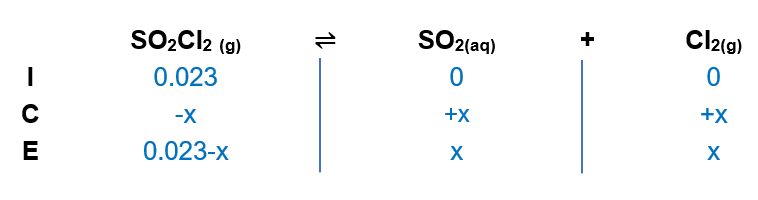# Problem: Consider the following reaction: SO2Cl2(g) ⇌ SO2(g) + Cl2(g)A reaction mixture is made containing an initial [SO2Cl2] left[ {{ m{SO}}_2 { m{Cl}}_2 } ight] of 2.3×10−2 M. At equilibrium, [Cl2] = 1.2×10−2 M. Calculate the value of the equilibrium constant (Kp).

###### FREE Expert Solution

(1) Setup an ICE chart:(2) Writing Kp expression where x = 1.2x10-2

88% (287 ratings)###### Problem Details

Consider the following reaction: SO2Cl2(g) ⇌ SO2(g) + Cl2(g)

A reaction mixture is made containing an initial [SO2Cl2] of 2.3×10−2 M. At equilibrium, [Cl2] = 1.2×10−2 M. Calculate the value of the equilibrium constant (Kp).